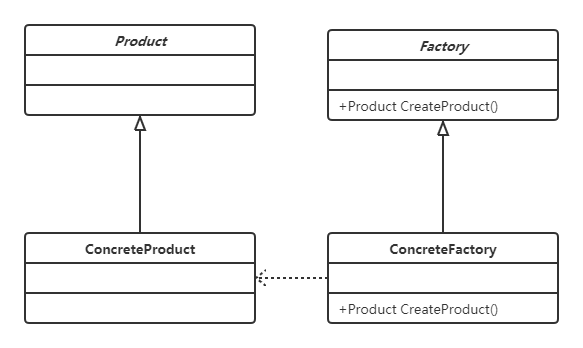# 从一个计算器开始说起——C#中的工厂方法模式

【Flutter实战】六大布局组件及半圆菜单案例

#### 举个例子

##### 第一个版本

• 取运算数
• 取运算符
• 输出结果
``````     class Program
{
static int GetOperatorIndex(string input)
{
int operatorIndex = 0;
for (; operatorIndex < input.Length; operatorIndex++)
{
if (!char.IsDigit(input[operatorIndex]))
break;
}
return operatorIndex;
}

static int GetOp(string input, int startIndex, int size = -1)
{
string subStr;
if (size == -1)
{
subStr = input.Substring(startIndex);
}
else
{
subStr = input.Substring(startIndex, size);
}
return int.Parse(subStr);
}

static int CalculateExpression(string input)
{
var operatorIndex = GetOperatorIndex(input); //得到运算符索引
var op1 = GetOp(input, 0, operatorIndex); //得到运算数1
var op2 = GetOp(input, operatorIndex + 1); //得到运算数2
switch (input[operatorIndex])
{
case '+':
return op1 + op2;
case '-':
return op1 - op2;
default:
throw new Exception("not support");
}
}

static void Main(string[] args)
{
string input = Console.ReadLine();
while(!string.IsNullOrEmpty(input))
{
var result = CalculateExpression(input);
Console.WriteLine("={0}", result);
input = Console.ReadLine();
}
}
}
``````

• 缺乏起码的抽象，至少加和减应该能抽象出操作类。
• 缺乏抽象造成了巨型客户端，所有的逻辑都嵌套在了客户端里面。
• 使用switch case缺乏扩展性，同时switch case也暗指了这部分代码是属于变化可能性比较高的地方，我们应该把它们封装起来。而且不能把他们放在和客户端代码一起

##### 工厂方法模式版本• 设计一个IOperator接口，对应抽象的Product
• 设计AddOperator和SubtractOperator，对应具体Product
• 设计IOperatorFactory接口生产Operator
• 设计OperatorFactory实现抽象IFactory

JAVA设计模式 1【创建型】设计模式介绍、单例模式的理解与使用

• IOperator接口
``````       interface IOperator
{
int Calculate(int op1, int p2);
}
``````
• 具体Operator
``````	class AddOperator : IOperator
{
public int Calculate(int op1, int op2)
{
return op1 + op2;
}
}

class SubtractOperator : IOperator
{
public int Calculate(int op1, int op2)
{
return op1 - op2;
}
}
``````
• Factory接口
``````	interface IOperatorFactory
{
IOperator CreateOperator(char c);
}
``````
• 具体Factory
``````	class OperatorFactory : IOperatorFactory
{
public IOperator CreateOperator(char c)
{
switch(c)
{
case '+':
return new AddOperator();
case '-':
return new SubtractOperator();
default:
throw new Exception("Not support");
}
}
}
``````
• 在CalculateExpression里面使用他们
``````   static IOperator GetOperator(string input, int operatorIndex)
{
IOperatorFactory f = new OperatorFactory();
return f.CreateOperator(input[operatorIndex]);
}

static int CalculateExpression(string input)
{
var operatorIndex = GetOperatorIndex(input);
var op1 = GetOp(input, 0, operatorIndex);
var op2 = GetOp(input, operatorIndex + 1);
IOperator op = GetOperator(input, operatorIndex);
return op.Calculate(op1, op2);
}
``````

• 容易变化的创建部分被工厂封装了起来，工厂和客户端以接口的形式依赖，工厂内部逻辑可以随时变化而不用担心影响客户端代码
• 工厂部分可以放在另外一个程序集，项目规划会更加合理
• 客户端仅仅需要知道工厂和抽象的产品类，不需要再知道每一个具体的产品（不需要知道如何构建每一个具体运算符），符合迪米特法则
• 扩展性增强，如果之后需要添加乘法multiple，那么仅需要添加一个Operator类代表Multiple并且修改Facotry里面的生成Operator逻辑就可以了，不会影响到客户端

##### 反射版本

• 定义一个描述属性以识别运算符
• 在运算符中添加该描述属性
• 在工厂启动的时候，扫描程序集以注册所有运算符

• 描述属性
``````    class OperatorDescriptionAttribute : Attribute
{
public char Symbol { get; }
public OperatorDescriptionAttribute(char c)
{
Symbol = c;
}
}
``````
• 添加描述属性到运算符
``````    [OperatorDescription('+')]
class AddOperator : IOperator
{
public int Calculate(int op1, int op2)
{
return op1 + op2;
}
}

[OperatorDescription('-')]
class SubtractOperator : IOperator
{
public int Calculate(int op1, int op2)
{
return op1 - op2;
}
}
``````
• 让工厂使用描述属性
``````    class OperatorFactory : IOperatorFactory
{
private Dictionary<char, IOperator> dict = new Dictionary<char, IOperator>();
public OperatorFactory()
{
Assembly assembly = Assembly.GetExecutingAssembly();
foreach (var type in assembly.GetTypes())
{
if (typeof(IOperator).IsAssignableFrom (type)
&& !type.IsInterface)
{
var attribute = type.GetCustomAttribute<OperatorDescriptionAttribute>();
if(attribute != null)
{
dict[attribute.Symbol] = Activator.CreateInstance(type) as IOperator;
}
}
}
}
public IOperator CreateOperator(char c)
{
if(!dict.ContainsKey(c))
{
throw new Exception("Not support");
}
return dict[c];
}
}
``````

``````  [OperatorDescription('*')]
class MultipleOperator : IOperator
{
public int Calculate(int op1, int op2)
{
return op1 * op2;
}
}
``````